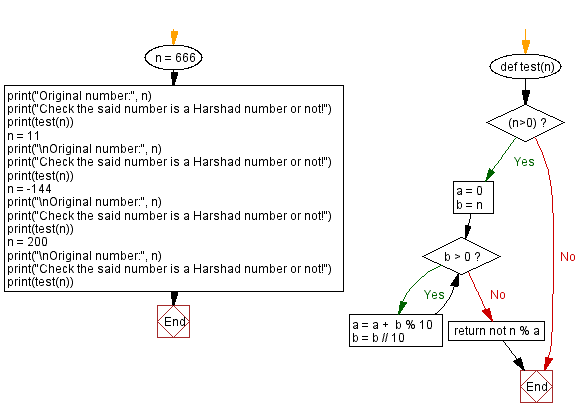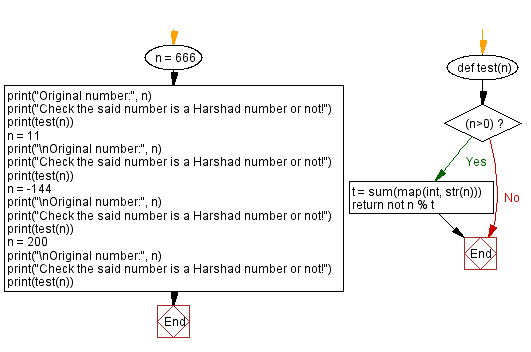﻿ Python - Check if a number is a Harshad number or not

# Python Exercises: Check if a number is a Harshad number or not

## Python Math: Exercise-90 with Solution

In recreational mathematics, a Harshad number in a given number base, is an integer that is divisible by the sum of its digits when written in that base.
Example: The number 18 is a Harshad number in base 10, because the sum of the digits 1 and 8 is 9 (1 + 8 = 9), and 18 is divisible by 9.
The number 19 is not a harshad number in base 10, because the sum of the digits 1 and 9 is 10 (1 + 9 = 10), and 19 is not divisible by 10.
Harshad numbers in base 10 form the sequence:
1, 2, 3, 4, 5, 6, 7, 8, 9, 10, 12, 18, 20, 21, 24, 27, 30, 36, 40, 42, 45, 48, 50, 54, 60, 63, 70, 72, 80, 81, 84, 90, 100, 102, 108, 110, 111, 112, 114, 117, 120, 126, 132, 133, 135, 140, 144, 150, 152, 153, 156, 162, 171, 180, 190, 192, 195, 198, 200, ....

Write a Python program to check if a given number is a Harshad number or not. Return True if the number is Harshad otherwise False.

Sample Data:
(666) -> True
(11) -> False
(-144) -> None
(200) -> True

Sample Solution-1:

Python Code:

``````def test(n):
if (n>0):
a = 0
b = n
while b > 0:
a = a +  b % 10
b = b // 10
return not n % a

n = 666
print("Original number:", n)
print("Check the said number is a Harshad number or not!")
print(test(n))
n = 11
print("\nOriginal number:", n)
print("Check the said number is a Harshad number or not!")
print(test(n))
n = -144
print("\nOriginal number:", n)
print("Check the said number is a Harshad number or not!")
print(test(n))
n = 200
print("\nOriginal number:", n)
print("Check the said number is a Harshad number or not!")
print(test(n))
```
```

Sample Output:

```Original number: 666
Check the said number is a Harshad number or not!
True

Original number: 11
Check the said number is a Harshad number or not!
False

Original number: -144
Check the said number is a Harshad number or not!
None

Original number: 200
Check the said number is a Harshad number or not!
True
```

Flowchart:## Visualize Python code execution:

The following tool visualize what the computer is doing step-by-step as it executes the said program:

Sample Solution-2:

Python Code:

``````def test(n):
if (n>0):
t = sum(map(int, str(n)))
return not n % t
n = 666
print("Original number:", n)
print("Check the said number is a Harshad number or not!")
print(test(n))
n = 11
print("\nOriginal number:", n)
print("Check the said number is a Harshad number or not!")
print(test(n))
n = -144
print("\nOriginal number:", n)
print("Check the said number is a Harshad number or not!")
print(test(n))
n = 200
print("\nOriginal number:", n)
print("Check the said number is a Harshad number or not!")
print(test(n))
```
```

Sample Output:

```Original number: 666
Check the said number is a Harshad number or not!
True

Original number: 11
Check the said number is a Harshad number or not!
False

Original number: -144
Check the said number is a Harshad number or not!
None

Original number: 200
Check the said number is a Harshad number or not!
True
```

Flowchart:## Visualize Python code execution:

The following tool visualize what the computer is doing step-by-step as it executes the said program:

Python Code Editor:

Have another way to solve this solution? Contribute your code (and comments) through Disqus.

Previous Python Exercise: Check a number is a repdigit number or not.
Next Python Exercise: Next number containing only distinct digits.

What is the difficulty level of this exercise?

Test your Programming skills with w3resource's quiz.

﻿

## Python: Tips of the Day

Summing a sequence of numbers (calculating the sum of zero to ten with skips):

```>>> l = range(0,10,2)
>>> sum(l)
20
```

We are closing our Disqus commenting system for some maintenanace issues. You may write to us at reach[at]yahoo[dot]com or visit us at Facebook